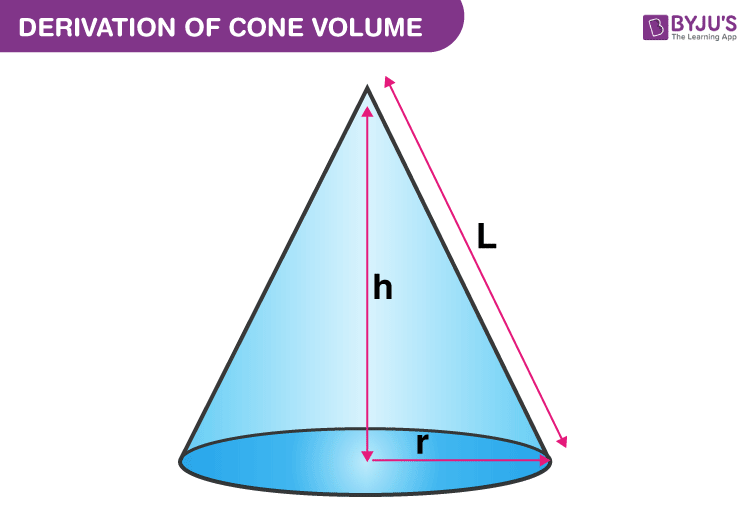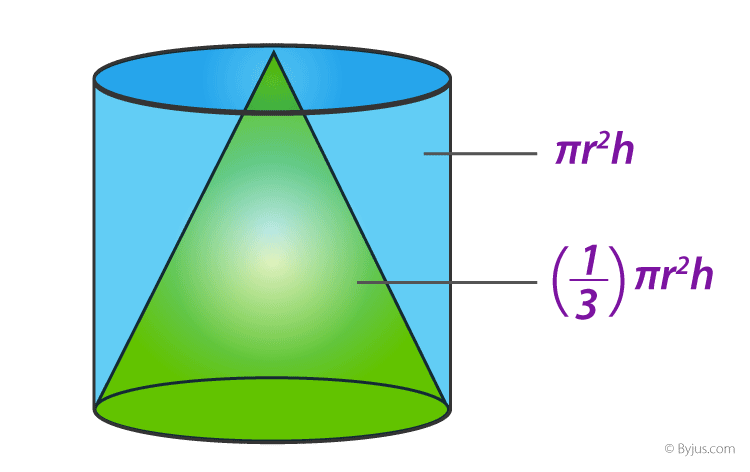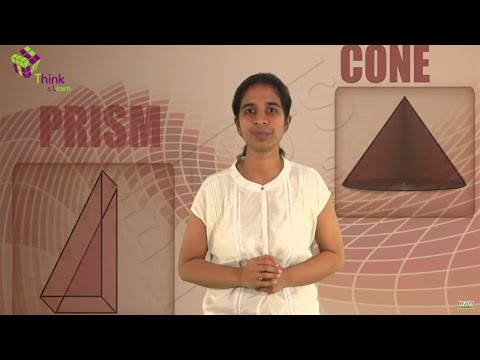Jet Set Go! All about Aeroplanes Jet Set Go! All about Aeroplanes

# Volume of a Cone

The volume of a cone defines the space or the capacity of the cone. A cone is a three-dimensional geometric shape having a circular base that tapers from a flat base to a point called apex or vertex. A cone is formed by a set of line segments, half-lines or lines connecting a common point, the apex, to all the points on a base that is in a plane that does not contain the apex.

A cone can be seen as a set of non-congruent circular disks that are stacked on one another such that the ratio of the radius of adjacent disks remains constant.

## Volume of a Cone Formula

In general, a cone is a pyramid with a circular cross-section. A right cone is a cone with its vertex above the center of the base. It is also called right circular cone. You can easily find out the volume of a cone if you have the measurements of its height and radius and put it into a formula.Therefore, the volume of a cone formula is given as

The volume of a cone = (1/3) πr2h cubic units

Where,

‘r’ is the base radius of the cone

‘l’ is the slant height of a cone

‘h’ is the height  of the cone

As we can see from the above cone formula, the capacity of a cone is one-third of the capacity of the cylinder. That means if we take 1/3rd of the volume of the cylinder, we get the formula for cone volume.

Note: The formula for the volume of a regular cone or right circular cone and the oblique cone is the same.

 Related Links Volume Of Cube Cuboid Cylinder Volume of Sphere Volume of Pyramid Volume of Cuboid

### Derivation of Cone Volume

You can think of a cone as a triangle which is being rotated about one of its vertices. Now, think of a scenario where we need to calculate the amount of water that can be accommodated in a conical flask. In other words, calculate the capacity of this flask. The capacity of a conical flask is basically equal to the volume of the cone involved. Thus, the volume of a three-dimensional shape is equal to the amount of space occupied by that shape. Let us perform an activity to calculate the volume of a cone.Take a cylindrical container and a conical flask of the same height and same base radius. Add water to the conical flask such that it is filled to the brim. Start adding this water to the cylindrical container you took. You will notice it doesn’t fill up the container fully. Try repeating this experiment for once more, you will still observe some vacant space in the container. Repeat this experiment once again; you will notice this time the cylindrical container is completely filled. Thus, the volume of a cone is equal to one-third of the volume of a cylinder having the same base radius and height.

Now let us derive its formula. Suppose a cone has a circular base with radius ‘r’ and its height is ‘h’.  The volume of this cone will be equal to one-third of the product of the area of the base and its height. Therefore,

V = 1/3 x Area of Circular Base x Height of the Cone

Since, we know by the formula of area of the circle, the base of the cone has an area (say B) equals to;

B = πr2

Hence, substituting this value we get;

V = 1/3 x πr2 x h

Where V is the volume, r is the radius and h is the height.

## Solved Examples

Q.1: Calculate the volume if r= 2 cm and h= 5 cm.

Solution:

Given:

r = 2

h= 5

Using the Volume of Cone formula

The volume of a cone = (1/3) πr2h cubic units

V= (1/3) × 3.14 × 2×5

V= (1/3) × 3.14 × 4 ×5

V= (1/3) × 3.14 × 20

V = 20.93 cm3

Therefore, the volume of a cone = 20.93 cubic units.

Q.2: If the height of a given cone is 7 cm and the diameter of the circular base is 6 cm. Then find its volume.

Solution: Diameter of the circular base = 6 cm.

So, radius = 6/2 = 3 cm

Height = 7 cm

By the formula of cone volume, we know;

V = 1/3 πr2h

So by putting the values of r and h, we get;

V = 1/3 π 37

Since π = 22/7

Therefore,

V = 1/3 x 22/7 x 3x 7

V = 66 cu.cm.## Practice Worksheet on Volume of Cone

1. The height of a cone is 24 cm and the diameter of its base is 14 cm. Find the volume of the cone.
2. The height and the slant height of a cone are 21 cm and 28 cm respectively. Find the volume of the cone.
3. A semicircular sheet of diameter 32 cm is folded to form a conical cup. Find the capacity of the cup.

Stay tuned with BYJU’S – The Learning App to learn interesting maths-related articles and also watch engaging videos to learn with ease.

## Frequently Asked Questions on Volume of Cone

### What is the formula for the volume of a cone?

The formula for the volume of a cone is ⅓ 𝜋r2h cubic units, where r is the radius of the circular base and h is the height of the cone.

### How many times the volume of a cylinder is equal to the volume of a cone?

One-third of the volume of a cylinder is equal to the volume of a cone, having the same radius and height.

### What is the formula for the slant height of a cone?

The slant height of a cone l = √(h2 + r2), where h is the height of the cone and r is the radius of the circular base.

### What is the formula for the total surface area of a cone?

The total surface area of a cone is given by 𝜋r(l + r) square units, where r is the radius of the circular base and l is the slant height of the cone.

### What is the formula for the curved surface area of a cone?

The curved surface area of a cone is given by 𝜋rl square units, where r is the radius of the circular base and l = √(h2 + r2) is the slant height of the cone.

### How to find the volume of a cone, if its height and diameter are given?

We know that the volume of a cone = (1/3)πr2h cubic units
Since r = d/2, the volume of a cone becomes
V = (1/3)π(d/2)2h cubic units
V = (1/12)πd2h cubic units.
Hence, the formula for the volume of a cone is (1/12)πd2h cubic units, if its height and diameter are given.

### What will be the volume of a cone if its radius and height are doubled?

If r = 2r and h = 2h, then the volume of a cone is given as:
Volume of a cone = (1/3)π(2r)2(2h) cubic units
V = (⅓)π(4r2)(2h)
V = (8/3)πr2h
Thus, the volume of a cone becomes (8/3)πr2h, when its radius and height are doubled.

### What is the base surface area of a cone?

The base surface area of a cone is a circle, which equals πr2.

Test your Knowledge on Volume of a Cone